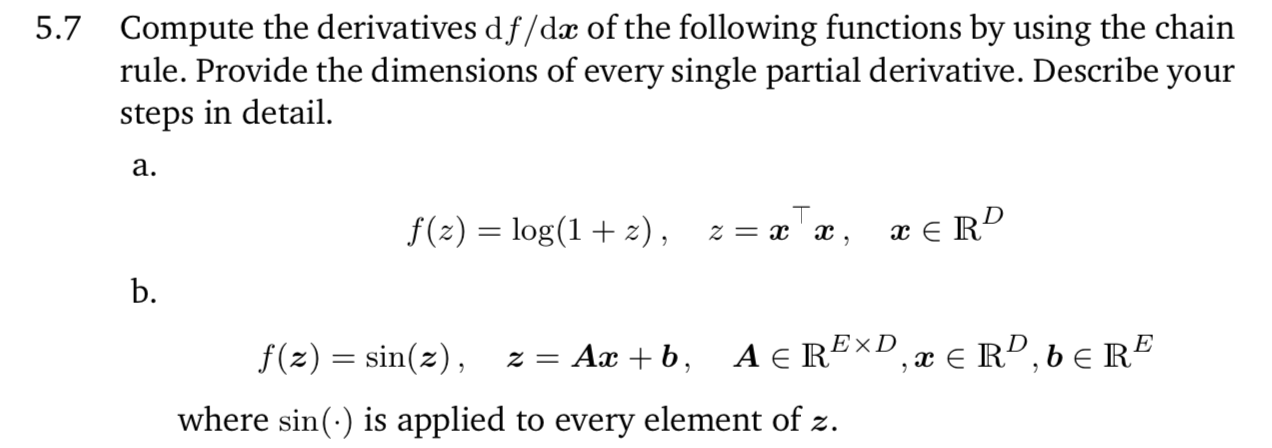### DS4420¶

The joy of autograd; a brief primer on pytorch for automatic differentiation.

Consider this exercise from the math for machine learning (MML) book.On the board we worked this out manually:

$\frac{2x}{1+z}$ = $\frac{2x}{1+x^tx}$

But this would get tedious; fortunately modern libraries use auto-diff. In this class you'll want to get familiar with at least one such library (the obvious two choices being pytorch and TensorFlow; I have a personal bias for the former).

In :
import numpy as np
import torch
import torch.optim as optim
import torch.nn as nn

In :
D = 10 # arbitrary dimension.
print(x)

tensor([0.1801, 0.3444, 0.4006, 0.6215, 0.2568, 0.3863, 0.5832, 0.4745, 0.8343,

In :
z = torch.dot(x, x)
f = torch.log(1 + z)


So from our manual result above, we should end up with: $\frac{2x}{1 + z}$

In :
(x / (1 + z))*2

Out:
tensor([0.1134, 0.2168, 0.2522, 0.3913, 0.1617, 0.2432, 0.3672, 0.2987, 0.5252,
0.0310], dtype=torch.float64, grad_fn=<MulBackward0>)

By taking a backward pass (the terminology comes from backprop, which we will discuss in some detail in later lectures), we collect the gradient automatically.

In :
f.backward()

In :
print(x.grad)

tensor([0.1134, 0.2168, 0.2522, 0.3913, 0.1617, 0.2432, 0.3672, 0.2987, 0.5252,
0.0310], dtype=torch.float64)


#### and now (b)¶

${\text{cos}}(Ax + b) \cdot A$

In :
D = 5 # arbitrary dimension.
print(x)

tensor([0.0143, 0.9790, 0.6805, 0.8771, 0.0953], dtype=torch.float64,

In :
E = 10
A = torch.tensor(np.random.random((E, D)))
A

Out:
tensor([[0.9670, 0.5487, 0.0196, 0.4444, 0.3262],
[0.3794, 0.7918, 0.9998, 0.0907, 0.8979],
[0.0238, 0.1552, 0.9408, 0.4540, 0.7213],
[0.0461, 0.6931, 0.9383, 0.2263, 0.5735],
[0.1622, 0.9763, 0.6564, 0.8489, 0.6759],
[0.1780, 0.4534, 0.0268, 0.5234, 0.3507],
[0.1702, 0.2508, 0.9425, 0.4099, 0.5830],
[0.7704, 0.2154, 0.5592, 0.2581, 0.9374],
[0.5028, 0.6831, 0.5410, 0.7700, 0.9633],
[0.1757, 0.9128, 0.7975, 0.6231, 0.2041]], dtype=torch.float64)
In :
b = torch.tensor(np.random.random((E)))
print(b)

tensor([0.2230, 0.8327, 0.9930, 0.4835, 0.0355, 0.7217, 0.7977, 0.3526, 0.9574,
0.4611], dtype=torch.float64)

In :
z = torch.mv(A, x) + b
f = torch.sin(z)

In :
torch.sin(z)

Out:
tensor([0.9350, 0.6309, 0.7764, 0.8853, 0.7785, 0.9942, 0.8621, 0.9553, 0.3643,
0.6254], dtype=torch.float64, grad_fn=<SinBackward>)
In :
z.shape

Out:
10
In :
# this is a bit funny but basically if we just call backward'
# implicitly Torch takes as loss.backward(torch.Tensor())'
f.backward(torch.Tensor(np.ones(z.shape)))

Out:
tensor([-0.5727, -2.9134, -3.6513, -2.2281, -2.8390], dtype=torch.float64)

How does this compare to our bespoke solution?

In :
torch.matmul( torch.cos(torch.mv(A, x) + b), A)

Out:
tensor([-0.5727, -2.9134, -3.6513, -2.2281, -2.8390], dtype=torch.float64,
grad_fn=<SqueezeBackward3>)

OK all is sane!

In [ ]: